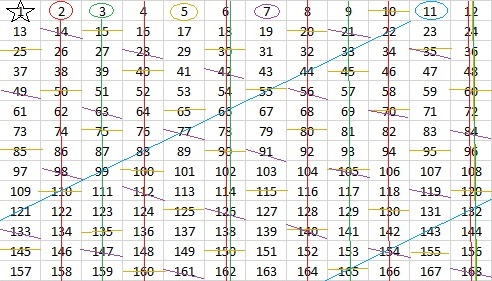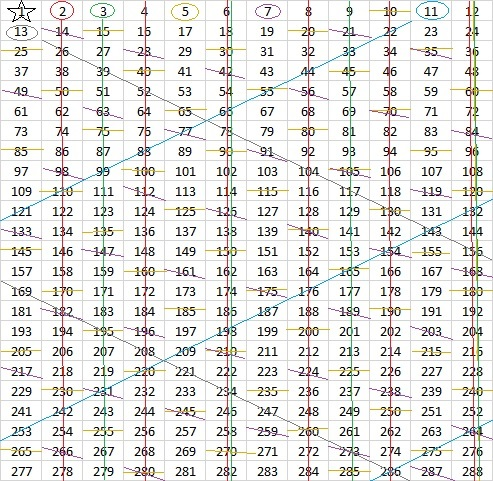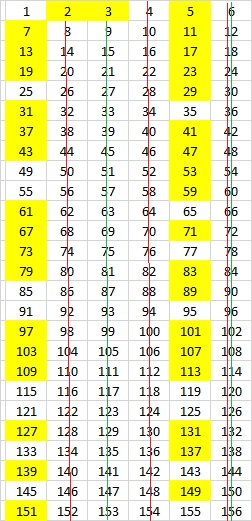# 1368 Playing with the Sieve of Eratosthenes

What if we didn’t use ten numbers across for our Sieve of Eratosthenes?

For example, 36 × 38 = 1368. We could make a Sieve of Eratosthenes writing 36 numbers across the grid and 38 numbers down. The last number on the grid would be 1368, and we could find all the prime numbers less than 1369 (which is 17²) by crossing out all the multiples of the prime numbers that appear on the top row. The trouble is that 36 numbers across makes a very big grid. Crossing out multiples of 2, 3, 5, and 7 will be very quick, but crossing out all the multiples of 11, 13, 17, 19, 23, 29, and 31 will not be so fun.

Grids that make use of the fact that (n-1)(n+1) = n²-1 can always give us a perfect rectangle and we will only need to cross out the multiples of the prime numbers in the top row to find ALL the prime numbers in any (n-1)×(n+1) list of numbers.

Here’s a 7 × 9 grid:Since it was 7 across, it was very easy to cross out all the multiples of 7. The multiples of 2 and 3 weren’t too difficult to find either, but the pattern for the multiples of 5 was not quite as nice. Fortunately, it is easy to spot those multiples, no matter how big a number they are.

Still, the first prime number on the second row is 11, so we should be able to go almost up to 11² = 121 on our grid:I couldn’t fit 120 on the grid without ruining the rectangle, but here’s a grid using 12 numbers across. Since 12 × 14 = 168 which is one less than 13², we can find all the prime numbers in the list simply by crossing out the multiples of the prime numbers in the top row.But the next number, 13, is only one number more than 12, and all of its multiples are staring at me making me feel very uncomfortable. It will be very easy to cross out all of the multiples of 13. That means we can extend the list of numbers to one less than the next prime number squared, which is 289 – 1 = 288. This time we get a perfect rectangle because 288 is also a multiple of 12:All of the circled numbers in the top row and every number that has not been crossed out below the top row are prime numbers.

Someone long ago figured out that if we make the grid six numbers across, all the prime numbers except 2 and 3 will appear in the same two columns, no matter how long the grid is:Every prime number greater than 3 is either one less or one more than a multiple of 6.

Since we always cross out the multiples of 2 anyway, what would happen if we didn’t include them in the grid at all?

Here is a grid with ten numbers across, but only odd numbers are included. Because 5 is a factor of 10, it is very easy to cross out all of the 5’s. Also, since 9 is one less than 10 and 11 is one more than 10, it is also easy to cross out all the multiples of 3 and 11. Crossing out the 7’s and the 19’s wasn’t too bad, either, but the 13’s and 17’s were not as fun.In my next post, I will share my favorite size of grid and the method I use to find all of the prime numbers on it. No prime numbers get circled in my method.

Some of the numbers in the grids had several lines through them.
If we made the 36 × 38 grid I mentioned at the beginning of the post, how many lines would 1368 have going through it?  After all, 1368 has 24 factors. What do you think?

Only three lines. One each for its prime factors, 2, 3 and 19.

Here’s more about the number 1368:

• 1368 is a composite number.
• Prime factorization: 1368 = 2 × 2 × 2 × 3 × 3 × 19, which can be written 1368 = 2³ × 3² × 19
• 1368 has at least one exponent greater than 1 in its prime factorization so √1368 can be simplified. Taking the factor pair from the factor pair table below with the largest square number factor, we get √1368 = (√36)(√38) = 6√38
• The exponents in the prime factorization are 3, 2, and 1. Adding one to each exponent and multiplying we get (3 + 1)(2 + 1)(1 + 1) = 4 × 3 × 2 = 24. Therefore 1368 has exactly 24 factors.
• The factors of 1368 are outlined with their factor pair partners in the graphic below.Here’s one of the MANY possible factor trees for 1368:This site uses Akismet to reduce spam. Learn how your comment data is processed.